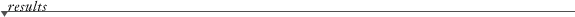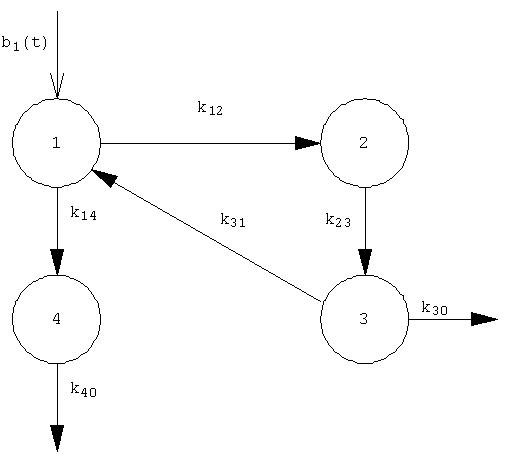Compartmental Modeling. Constant rate transfers
(Updated: 2021-12-12. Comments to: guillermo2046(at)gmail.com writing in the Subject:"BIOKMOD")

Here is applied SysModel package for solving linear compartmental model. The "compartmental matrix" shall be written: {{1,2,k12},...{i,j,kij},...,{n,n,knn}}, where kij is the rate transfer constant -clearance- from compartment i to compartment j. The radioactive decay constant, if it available , will be written in the same units that the rate transfer constans. All units mush be coherent with kij, so if kij is in days^-1 the time t to be evaluated the retencion must be in days.

 Enter the compartmental matrix: {{1, 2, 0.83}, {1, 4, 1.94}, {2, 3, Log/80}, {3, 0, 0.01155}, {3, 1, 0.0462}, {4, 0, 12}} Number of compartments:    Decay constant:   Initial conditions at time t = 0: Input function in each compartment : All values of this field must be {0,.., 0} if it is an impulsive single-input, because the inputs are the initial conditions. Time t to evaluate the content in each compartment (i.e.: t or {5, 20, 30}) : Range of t to be plotted:   From t-min   to t-max Time t, in days (kij in days^-1), to compute the acumulated disintegrations in each compartment :Differential equationSolutionPlot

Us (disintegrations in each compartment during a time t)

The values included by default represents the iodine biokinetic model -see the figure - where compartment 1 is the blood, compartment 2 is the thyroid , compartment 3 is the rest of the body, and "0" mean "out of the system" . The rate transfer values (clearance), in days^-1, taken from ICRP 78 are k10 = 1.9404, k12= 0.8316, k23 = Log/80, k30 = 0.01155 and k31= 0.0462, k30 = 0.01155 and k40 = 12. Them the compartmental matrix is defined:{{1, 2, 0.83}, {1, 4, 1.94}, {2, 3, Log/80}, {3, 0, 0.01155}, {3, 1, 0.0462}, {4, 0, 12}} . Also it assumed the radioactive decay constant of I-131 that is Log/8.02 (days^-1). It assumed a continuouos input "1.2 Exp[-0.2 t]" in compartment 1, then the input is written: {1.2 Exp[-0.2 t], 0, 0, 0}. Also it is supossed as initial contition {1, 0, 0, 0}.